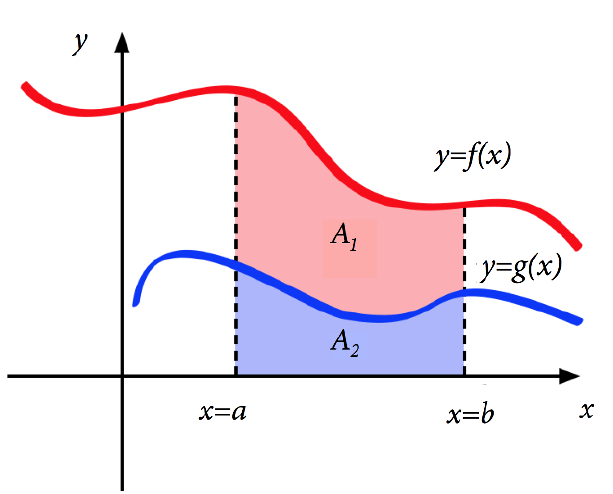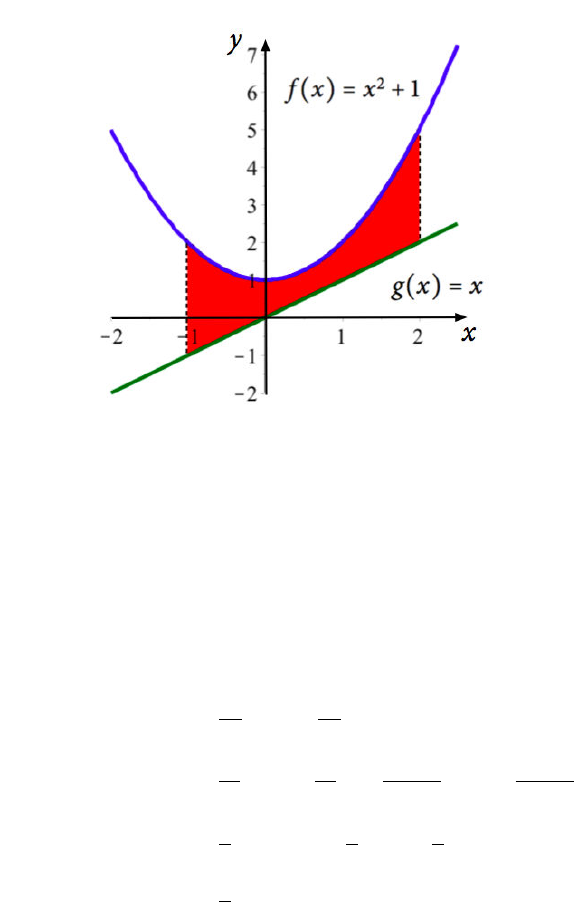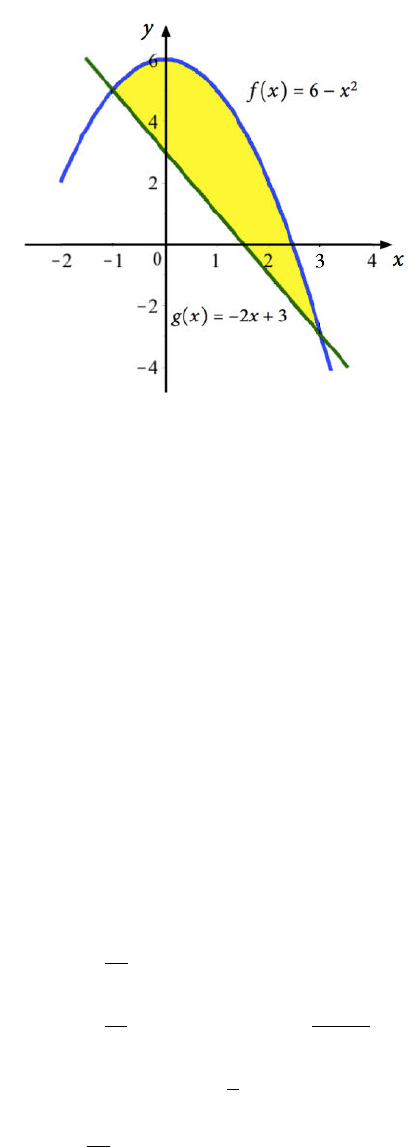Study Guides (400,000)
US (230,000)
NCSU (600)
MA (50)
MA 141 (40)
Final

# MA 141 Lecture 26: Area Between Two CurvesExam

Department
Mathematics
Course Code
MA 141
Professor
Kevin Flores
Study Guide
Final

This preview shows pages 1-2. to view the full 8 pages of the document.MA 141 Chapter 5
Section 5.1: Area Between Two Curves
In Chapter 4, we developed deﬁnite integrals to calculate the area "under" a curve - between
the graph of a function and the x-axis. We may want to ﬁnd the area of a more complicated
shape, such as the area between two curves.
Figure 1
From the picture, we can see that the area under the curve y=f(x)is given by
Zb
a
f(x)dx =A1+A2
while the area under the curve y=g(x)is given by
Zb
a
g(x)dx =A2.
If we wanted to ﬁnd just the area between the two curves, A1, we could do the following:
(A1+A2)A2=Zb
a
f(x)dx Zb
a
g(x)dx
=Zb
a
(f(x)g(x))dx
This yields the formula we will use to ﬁnd the area "between two curves".
1

Only pages 1-2 are available for preview. Some parts have been intentionally blurred.Ex: Find the area of the region bounded by f(x) = x2+ 1,g(x) = x,x=1and x= 2.
For any area problem like this, we should graph the functions.
From the graph, we can see that f(x) = x2+ 1 is greater than g(x) = xon the interval
[1,2].
Then the area we want is given by
Z2
1
(f(x)g(x))dx =Z2
1
(x2+ 1 x)dx
=x3
3+xx2
2
2
1
=23
3+ 2 22
2(1)3
31(1)2
2
=8
3+ 2 2 + 1
3+ 1 + 1
2
=9
2

Unlock to view full version

Only pages 1-2 are available for preview. Some parts have been intentionally blurred.Ex: Find the area of the region bounded by f(x) = 6 x2and g(x) = 2x+ 3.
Notice we are not given an interval here. Let’s graph to see if we can tell why.
The region "bounded" by the two curves is the region between their two intersection points.
So, we will ﬁnd the intersection points and use those for our bounds.
6x2=2x+ 3
0 = x22x3 = (x3)(x+ 1)
Then the bounds of our interval are x=1and x= 3.
We also need to know which function has the bigger values on this interval. We can see from
the graph that f(x) = 6 x2is bigger than g(x) = 2x+ 3 on the interval [1,3].
Z3
1
(f(x)g(x))dx =Z3
1
(6 x2(2x+ 3))dx
=Z3
1
(x2+ 2x+ 3)dx
=x3
3+x2+ 3x
3
1
=33
3+ 32+ 3(3) (1)3
3+ (1)2+ 3(1)
=9 + 9 + 9 1
31 + 3
=32
3